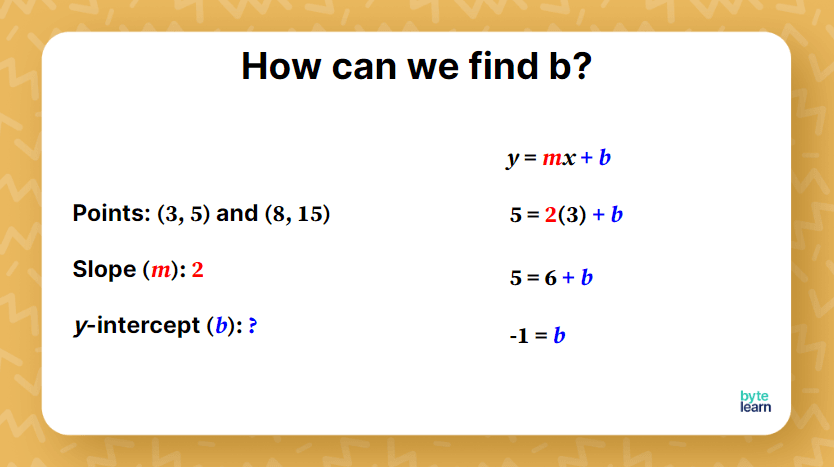ResourcesTestimonials
Plans
ResourcesTestimonials
PlansLesson plan

# Write an Equation From Two Points Lesson plan - 8th Grade Math

## Overview

In this lesson, students will learn how to write a linear equation from two points. Students will first review how to find slope and write in slope-intercept form. Students will then walk through writing an equation from two points using the knowledge they already know. With additional practice, you can expect this lesson to take one `45`-minute class period.

Linear Relationships And Functions
8.F.B.4

## Objective

Students will be able to write a linear equation from two points.

## Materials

• Teacher slideshow
• Online Practice

## How to Teach Writing Equations From Two Points

### Review of slope-intercept form

To begin the lesson, students should try a quick review of the slope-intercept form and find the slope from two points.Let students know that they will need to use their knowledge of slope-intercept form and slope from two points for writing equations from two points. Be sure to check in with students who seem to be struggling to try and catch misconceptions. These will likely be the students that will need your support most today.

### Finding the `y`-intercept

Show them this slide where you give them the equation in slope-intercept form, the two points, and the slope. Ask students to jot down ideas on how we can find the `y`-intercept.

Give students the time to share these ideas with the class. It is quite likely that students will together come up with the idea of substituting and solving for `b`.  Students might have used either of the two points to find the `b`-intercept. Discuss whether it makes a difference which point you pick.

Formalize the process by showing this slide and ask them to make notes.It is important that students are mindful of where they plug in each value since `y` should be isolated on the left side.

Because students have been accustomed to using slope-intercept form to identify the slope and `y`-intercept, some students may struggle with applying it to solve for the `y`-intercept algebraically.

### Writing the equation

Ask students to write the equation in slope-intercept form. Some students may be tempted to plug in an ordered pair as they did for finding the `y`-intercept. Remind them that they are writing a generalized equation that can work for any `x` and `y` for a point on the line.

### Review the steps for writing an equation from two points

Consider having the students reflect on the steps they took since the start of the class to write the equation for the points `(3,5)` and `(8,15)`. It may be beneficial to allow students to write informal steps for themselves. Then, students can share their steps with their peers to help deepen student understanding. Share this slide for those who want to take more formal notes.

### Class practice

Based on discussions from the previous example, students should understand the steps they will need to take to write the equation of the line from two points. It may help to review the steps.

The first two class practice problems are scaffolded - you ask the students to find the slope and the `y`-intercept, and you give the slope-intercept form of the equation. The color coding will help students to see where the values should be plugged in.

After two practice problems, give them a problem that is not scaffolded. Encourage them to look at their notes.

Encourage students to choose the ordered pairs that seem the easiest for them to work with; however, also encourage students not to use the same combination that their shoulder partner uses. This problem is an excellent way for students to see that no matter which points they pick, the equation of the line in the slope-intercept form will stay the same.

Give students a chance to talk about why they chose specific ordered pairs, what areas of the problem they were successful with, and what areas of the problem they struggled with. Allowing students to reflect on their learning and the steps they have taken to solve a problem can help solidify what they have learned.

## Writing Equations From Two Points Practice

After you’ve completed the examples with the whole class, it’s time for some independent practice! ByteLearn gives you access to tons of practice problems for writing equations from two points. Check out the online practice and assign to your students for classwork and/or homework!Writing Equations From Two Points Practice
Problem 1 of 4
<p>What is the equation of the line that passes through the points `(10, 4)` and `(-5, -14)`.<br><highlight data-color="#666" data-style="italic">Write your answer in slope-intercept form using integers and/or simplified fractions.</highlight></p>

View this practice